# 2nd PUC Basic Maths Question Bank Chapter 13 Heights And Distances Ex 13.1

Students can Download Basic Maths Exercise 13.1 Questions and Answers, Notes Pdf, 2nd PUC Basic Maths Question Bank with Answers helps you to revise the complete Karnataka State Board Syllabus and score more marks in your examinations.

## Karnataka 2nd PUC Basic Maths Question Bank Chapter 13 Heights and Distances Ex 13.1

Part – A

2nd PUC Basic Maths Heights and Distances Ex 13.1 Two Marks Questions and Answers

Question 1.
The angle of elevation of the top of a tower at a distance 500 metres from its foot is 30°. Find the height of the tower.
Sol.
From the triangle ABC
B be the position of the observer & AC = height of the pole h.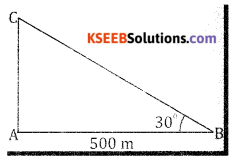tan 30° = $$\frac{\mathrm{AC}}{\mathrm{AB}}$$; $$\frac{1}{\sqrt{3}}=\frac{h}{500}$$
∴ h = $$\frac{500}{\sqrt{3}}=500 \sqrt{3}$$ Height of the tower m $$\frac{500 \sqrt{3}}{2} \mathrm{mts}$$

Question 2.
The angle of elevation of the top of a chimney at a distance of 100 metres from a foot is 30°. Find its height.
in triangle ABC we have B as the position of observer, AC height of tan 30° = $$\frac{A C}{A B}$$ the chimney = h$$\frac{1}{\sqrt{3}}=\frac{h}{100}$$
$$\mathrm{h}=\frac{100}{\sqrt{3}}=\frac{100 \sqrt{3}}{3} \mathrm{m}$$Question 3.
From a ship a mast head 40 meters high the angle of depression of a boat is observed to be 45°. Find its distance from the ship.
In triangle ABC, we have AC as mast head, AB is distance from the ship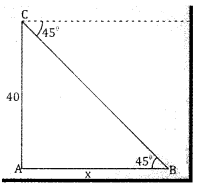tan 45° = $$\frac{A C}{A B}$$
$$1=\frac{40}{x}$$
⇒ x = 40
∴ Distance from the ship is 40 mts

Question 4.
What is the angle of elevation of the sun when the length of the shadow of a pole is $$\frac{1}{\sqrt{3}}$$ times the height of the pole?
Let the height of the pole be AC = h & length of the shadow = AB = $$\frac{1}{\sqrt{3}}$$ hFrom triangle ABC we have tan
tan θ = $$\frac{A C}{A B}$$ tan θ = $$\frac{\mathrm{h}}{\frac{1}{\sqrt{3}}}=\sqrt{3}$$ = tan 60°
⇒ θ = 30°

Question 5.
Find the angle of elevation of the sun when the shadow of a tower 75 meters high is meters $$25\sqrt{3}$$ long.
AC = height of the tower = h = 75 mts AB = Shadow of a tower = $$25\sqrt{3}$$ from triangle ABC, we have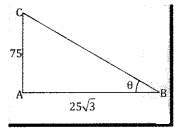tan θ = $$\frac{A C}{A B}=\frac{75}{25 \sqrt{3}}=\frac{3}{\sqrt{3}}=\sqrt{3}$$ = tan 60°
⇒ θ = 60°Question 6.
A kite flying at a height of h is tied to a thread which is 500m long. Assuming that there is no kink in the thread and it makes an angle of 30° with the ground. Find the height of the kite.
Let AC = height of the kite BC = 500 mts From ∆ ABC we havesin 30° = $$\frac{A C}{B C}=\frac{h}{500}$$
$$\frac{1}{2}=\frac{h}{500} \Rightarrow h=\frac{500}{2}=250 \mathrm{mts}$$
∴ the heights of the kite = 250 mts.

Question 7.
A ladder leaning against a wall makes an angle of 60° with the ground. The foot of the ladder is 6m away from the wall. Find the length of the ladder.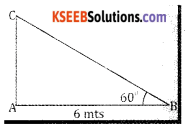Let AC be the ladder from triangle ABC we have
cos 60° = $$\frac{A B}{B C}=\frac{6}{B C}$$
$$\frac{1}{2}=\frac{6}{\mathrm{BC}} \Rightarrow \mathrm{BC}=12 \mathrm{mts}$$
∴ The length of the ladder = 12 mts.

Question 8.
Find the angle of elevation of the sun’s rays from a point on the ground at a distance of $$3 \sqrt{3}m$$ , from the foot of tower 3m high.Let AC be the height of the pole AC = 3mts & AB = $$3 \sqrt{3}m$$ mts From the triangle ABC we have
tan θ = $$\frac{A C}{A B}=\frac{3}{3 \sqrt{3}}=\frac{1}{\sqrt{3}}$$ = tan 30 ⇒ = 30°
∴ The angle of elevation is 30°Part – B

2nd PUC Basic Maths Heights and Distances Ex 13.1 Four or Five marks questions and answers

Question 1.
The angles of elevation of the top of a tower from the base and the top of a building are 60° and 45° The building is 20 meters high. Find the height of the tower.
Let AD be the tower & CE be the building. Let the distance between the tower and building be x in ∆ ABC,
tan 45° = $$\frac{A B}{B C}=\frac{h}{x}$$
$$1=\frac{\mathrm{h}}{\mathrm{x}} \Rightarrow \mathrm{x}=\mathrm{h}-(1)$$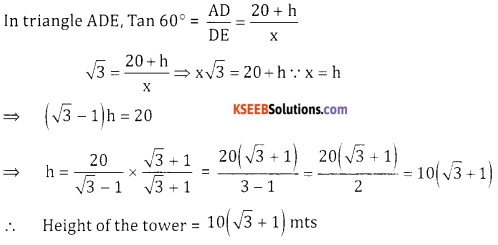Question 2.
The shadow of a tower standing on a level plane is found to be 50 meters longer when sun’s altitude is 30°. Than when it is 60°. Find the height of the tower.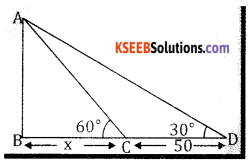Let AB be the tower In triangle ABC, tan 60° = $$\frac { h }{ x }$$
$$\sqrt{3}=\frac{h}{x}$$
$$h=\sqrt{3 x}$$
In triangle ABD, tan 30° = $$\frac{h}{50+x}$$
50 + x = $$\sqrt{3}$$
50 + x = $$\sqrt{3} \cdot \sqrt{3} x$$ ∵ h = $$\sqrt{3} x$$
50 = 3x – x ⇒ 2x = 50 ⇒ x = 25 mts
∴ Height of the tower h = 25$$\sqrt{3}$$ mtsQuestion 3.
An Aeroplane when flying at a heights of 2000 meters passes vertically above another plane at an instant, when their angles of elevation from the same point of observation are 60° and 45° respectively. Find the distance between the Aeroplanes.Let A & B are Aeroplanes Let h be the distance between the aeroplanes
From triangle ACDFrom triangle BCDQuestion 4.
From a point on the line joining the feet of two poles of equal heights, the angles of elevation of the tops of the poles are observed to be 30° and 60°. If the distance between the poles is a Find (i) the height of the poles (ii) the position of the point of observation.Let AB & DE are two poles∴ Height of the poles is $$\frac{\sqrt{3}}{4} a$$
Position of c from B is x = $$\sqrt{3 h}$$
∴ position of the point of observation = $$\frac{\sqrt{3} \cdot \sqrt{3} a}{4}=\frac{3 a}{4}$$Question 5.
The angles of elevation of the top of a tower from two points distant a and b (a < b) from its foot and the same straigth line from it are 30° and 60°. Show that the height of the tower is $$\sqrt{a b}$$.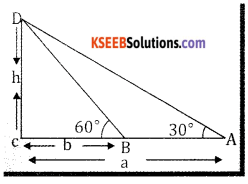Let CD is the tower from rt angled triangle ACD
we have tan 30 = $$\frac { h }{ a }$$ ⇒ h = $$\frac{a}{\sqrt{3}}$$ …(1)
From rt angled triangle BCD
tan 60° = $$\frac { h }{ b }$$
$$\sqrt{3}=\frac{h}{b} \Rightarrow h=\sqrt{3} b-(2)$$
From 1 & 2 we have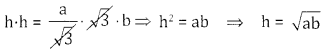Question 6.
A flag staff stands upon the top of a building. At a distance of 20 meters the angles of elevation of the top of the flag staff and building are 60° and 60° respectively. Find the height of the flag staff.Let BC = Building
AB = flagstaff
From triangle ACD we have
tan 60° = $$\frac{A C}{C D}=\frac{A B+B C}{20}=\sqrt{3}$$
AB + BC = 20 $$\sqrt{3}$$ …. (1)
In triangle BCD, tan 30° = $$\frac{B C}{C D} ; \quad \frac{1}{\sqrt{3}}=\frac{B C}{20}$$
BC = $$\frac{20}{\sqrt{3}}$$ …. (2)Question 7.
From the top of a cliff, the angles of depression of two boats in the same vertical plane as the observer are 30° and 45°. If the distance between the boats is 100 meters, find the height of the cliff.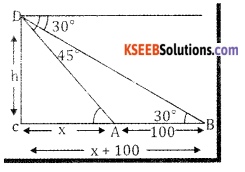Let the height of the cliff be h A & B are two boats
From triangle DCA
tan 45° = $$\frac{D C}{A C}=\frac{h}{x}$$
$$1=\frac{\mathrm{h}}{\mathrm{x}} \Rightarrow \mathrm{h}=\mathrm{x}$$ ….. (1)
From triangle BCDQuestion 8.
From a point A due north of the tower, the elevation of the top of the tower is 60°. From a point B due south, the elevantion is 45°, if AB = 100 metres. Show that the height of the tower is 50 $$\sqrt{3}(\sqrt{3}-1)$$ meters.A and B are positions of observation AB = 100 mts and CD is the height of tower and CD = h
From rt angled triangle ACD
tan 60° = $$\frac{\mathrm{CD}}{\mathrm{AD}}$$
$$\sqrt{3}$$ = $$\frac{h}{A D} \Rightarrow A D=\frac{h}{\sqrt{3}}$$ …..(1)

From rt angled triangle BCD
tan 45° = $$\frac{\mathrm{CD}}{\mathrm{BD}}$$
$$1=\frac{h}{B D} \Rightarrow B D=h$$ …. (2)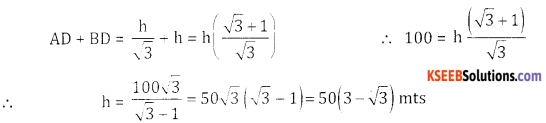Question 9.
A person at the top of a hill observes that the angles of depression of two consecutive kilometers stones on a road leading to the foot of the hill and in the same vertical plane containing the position of the observer are 30° and 60°. Find the height of the hill.
Let CD be the hill and CD = h mts
In ∆ ADC, we have tan 60° = $$\frac{C D}{A D} \Rightarrow \sqrt{3}=\frac{h}{x}$$
$$\Rightarrow \quad x=\frac{h}{\sqrt{3}} \Rightarrow h=\sqrt{3} x$$ …. (1)
From 1 and 2 we get
1 + x = $$\sqrt{3} \cdot \sqrt{3} x$$ (h = $$\sqrt{3} x$$)
1 + x = 3x
1 = 2x ⇒ x = 5 $$\frac{1}{2}$$
The height of the hill = h = $$\sqrt{3} x=\sqrt{3} \cdot \frac{1}{2}=\frac{\sqrt{3}}{2} \mathrm{mts}$$ mts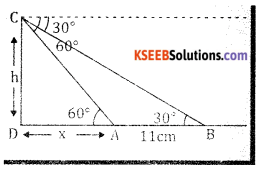Question 10.
The angles of elevation of the top of a tower from the base the top of a building are 60° & 45° the building is 32 meters high find the height of the towerLet CD is the tower
AB is the house = 32 meters
From the rt angled triangle ACE
tan 45° = $$\frac{C E}{A E}=\frac{C E}{B D}=1$$
⇒ CE = BD …. (1)
Again from rt angled triangle ABD
tan 30° = $$\frac{A B}{B D}=\frac{32}{B D}=\frac{1}{\sqrt{3}}$$
⇒ BD = $$32 \sqrt{3}$$
From 1 and 2 we get CE = $$32 \sqrt{3}$$ mts
∴ Height of the tower = h = DE + CE = 32 + $$32 \sqrt{3}$$ = -32(1 + $$\sqrt{3}$$)mtsQuestion 11.
The angle of elevation of a tower from a point on the ground is 30°. At a point on the horizontal line passing through the foot of the tower and 100 metres nearer it, the angle of elevation is found to be 60°. Find the height of the pole.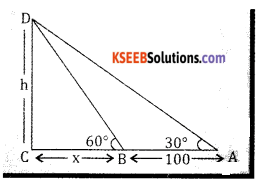Let CD = Tower
From a rt angled triangle ACD
tan 30° = $$\frac{\mathrm{CD}}{\mathrm{AC}}$$
\frac{1}{\sqrt{3}}=$$\frac{h}{100+x}$$
100+ x = $$\mathrm{h} \sqrt{3}$$ …. (1)

From triangle BCD
tan 60° = $$\frac{\mathrm{CD}}{\mathrm{CB}}$$
$$\sqrt{3}=\frac{h}{x} \Rightarrow h=\sqrt{3} x$$ …. (2)

From 1 & 2 we get
100 + x = $$\sqrt{3} \cdot \sqrt{3} x$$
100 = 3x – x ⇒ 2x = 100 ⇒ x = 50 :
∴ Height of the tower = h = $$\sqrt{3 x}$$ = $$50\sqrt{3}$$ mts
Hence Distance of the first point from the tower ₹ 100 + x = 100 + 50 = 150 mtsQuestion 12.
A Person is at the top of a tower 75 feet high from there he observes a vertical pole and finds the angles :: of depressions of the top and the bottom of the pole which are 30° and 60° respectively. Find the height of the pole.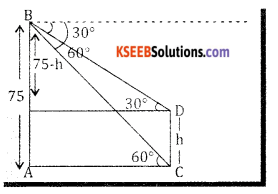Let AB = Tower, CD = Pole = h
From triangle ABC we get
tan 60° = $$\frac{A B}{A C} \Rightarrow \frac{75}{A C}=\sqrt{3}$$
⇒ AC = $$\frac{75}{\sqrt{3}} ….. (1)$$ …. (1)

From triangle BDE⇒ AC = $$\sqrt{3}(75-h)$$

From 1 and 2 we get
$$\frac{75}{\sqrt{3}}=\sqrt{3}(75-h) \Rightarrow 75=(\sqrt{3})^{2}(75-h)$$
75 = 225 – 3h 3h ⇒ 150 ⇒ h = 50
∴ Height of the pole -50ft

error: Content is protected !!